# How to Rationalize the Denominator of a Fraction

Knoji reviews products and up-and-coming brands we think you'll love. In certain cases, we may receive a commission from brands mentioned in our guides. Learn more.
This article shows you all the steps to rationalizing the denominator of a fraction that contains at least one square root term in the denominator into a new fraction, of equal value, that does not have a square root term in the denominator.

One of the typical problems encountered in elementary algebra is rationalizing the denominator. Recall that rationalizing the denominator means to convert the original fraction that contains at least one square root term in the denominator into a new fraction, of equal value, that does not have a square root term in the denominator. This article will take the reader through several simple steps that show how to rationalize the denominator for most common fraction types. There is, also, a formula section below each example that provides a detailed algebraic method used in deriving a formula wherein one can simply plug in the values of the original fraction into a new fraction with a rational denominator.

As the reader progresses through the seven major methods and their resulting final formulas, one will quickly discover that each subsequent fraction type will become more complicated to rationalize and the resulting fraction with a rational denominator more complicated in turn.

The mathematical level involved in this article is simple elementary algebra; however, as the fractions become more difficult, the steps will take longer to solve and result in tedious computations. With that being said, let us start with a simple fraction and progress forward:

#1) Rationalizing the denominator with a single square root in the denominator:

Given 1/√2 how does one solve it? The answer to solving every problem that contains a denominator that needs to be rationalized is to multiply the entire fraction by the equivalent value of one. Remember when a fraction is multiplied by one, the value of the fraction does not change. What changes is how the fraction “looks.” For example solving the above fraction we have: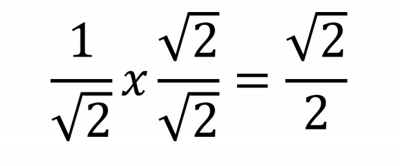As can be seen by the above example, the fraction was multiplied by the value of the existing denominator in the original fraction. In order not to change the value of the fraction, the fraction needed to be multiplied by the square root of two in both the denominator and numerator. This second fraction, called a modifying fraction, once multiplied to the original fraction converts the denominator of the original fraction into a term that does not contain a radical term (i.e. a square root term). The end result is a new fraction with the denominator rationalized while still maintaining the original value of the unmodified fraction.

This is the basic technique that will be applied over and over again to each type of fraction presented. The mathematical variations used to solve each subsequent problem will be slight.

The formula section will detail the algebraic method used to derive a simple plug-in formula used to rationalize these types of fractions. These formulas are useful to have handy especially if you will be encountering many such problems and do not have the time to rationalize each and every fraction encountered.

Formula for equation #1: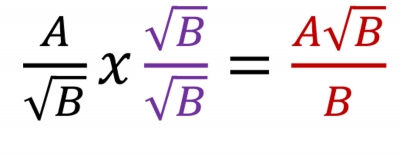#2) Rationalizing the denominator of a cubic, or higher, root.

The trick here is to realize that one must multiply the initial fraction in such a manner that the denominator has been completely rationalized. For example: If the denominator is a cubic root, root three, the fraction needs to be multiplied by itself twice. If the denominator is a 10th root, root 10, then it would need to be multiplied by itself nine times. In short, whatever value the root is, root “c,” the fraction needs to be multiplied (top and bottom) by itself one less times the value of root of the original fraction. That is it must be multiplied by itself root “c-1” times.In this case the root has a value of 9 then it needs to be multiplied by itself 8 times since (9 – 1 = 8).

Formula for equation #2: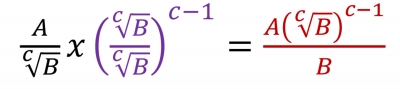#3) Rationalizing the denominator of a cubic, or higher, root that contains a squared, or higher, term inside the root:

This is a slight variation of the above example. Since the original fraction has a term that raised to a higher power than one, all one needs to do is find the difference between the power inside the radical and the root of the radical and use that difference found to multiply the modifying fraction by that difference.

For example: If the fraction has is fifth root and the term inside is a cubic, raised the third power, then the difference between 5 and 3 is 2. That is: 5 - 3 = 2. See example below: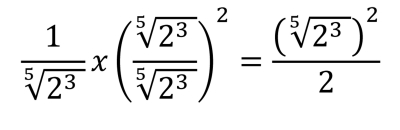Formula for equation #3 for c > d: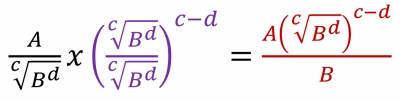#4) Solving fractions that contain two terms in the denominator: with one term being a square root.

To solve these types of fractions one needs to introduce the concept of the conjugate. The conjugate is a simple mathematical construct. A conjugate is simply the opposite of the addition or subtraction operation used when combining two terms. For example if you have 5 + 3 as the original set of terms then the conjugate would be written as 5 – 3. It is that simple! The advantage of multiplying a set of terms by its conjugate is that the resulting set of terms will be the difference of two squares. For example: (5+3) x (5-3)=(5x5 + (5x3 -5x3 this cancels)- 3x3).

Why would this be advantageous one might ask? The reason is simple: One wants to find a method to multiply the square root by itself thus eliminating the square root term!

Therefore the purpose of multiplying by the conjugate is to eliminate the square root. In all problems you will want the square root to be removed from the denominator of the fraction. If you start with a “+” between the two numbers you multiply the same two number with “-“ between them and vice versa.

The example below shows how to solve a fraction using a conjugate when you have a two terms one of which is square root in the denominator: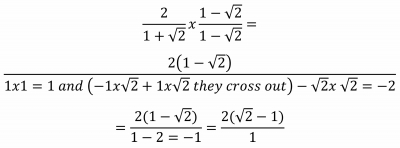Formula for equation #4: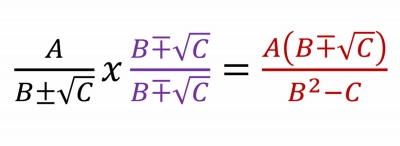#5) Using a conjugate when you have two square roots in the denominator: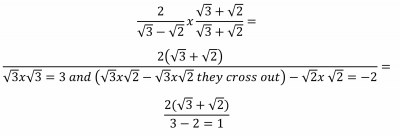Formula for equation #4: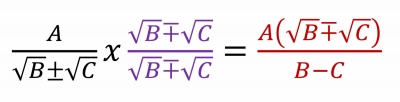#6) Using a conjugate when you have three terms in the denominator which two of them are two separate square roots:

In solving this problem, group any two terms as if they were a single term. Then multiply the conjugate between the two terms acting as one and the third term in the original fraction. See example below:

Step 1: Multiply by the conjugate (note the first two terms were grouped together):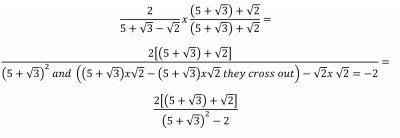Step 2: Expand the term that is squared and consolidate the resulting results: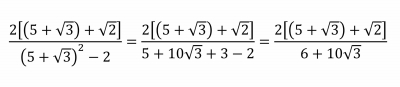Step 3: Multiply by the conjugate again: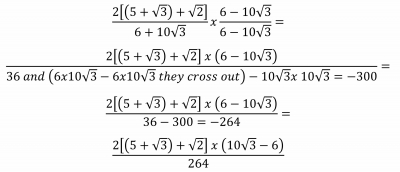Formula for equation #5: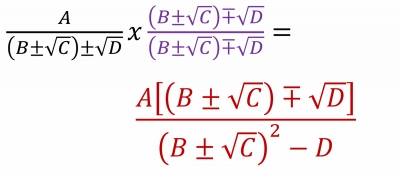Expanding the denominator: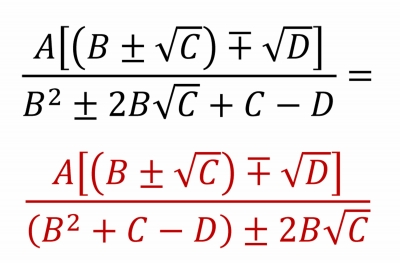Using the conjugate again we get formula for equation #6: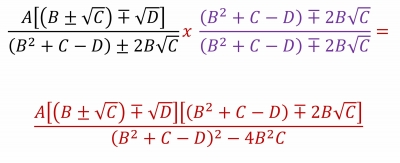#7) Rationalizing the denominator when it has 3 square root terms: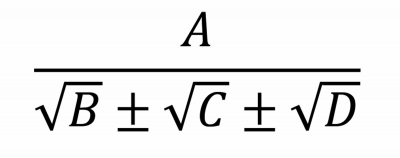Step 1: Multiply by the conjugate (again pick two terms to act as one):Step 2: Expand the term that is squared and consolidate the results: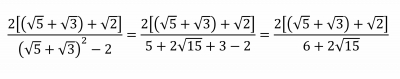Step 3: Multiply by the conjugate again: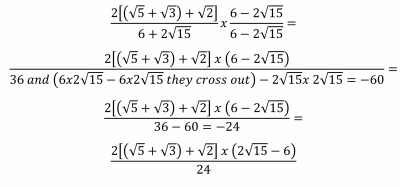Deriving the formula: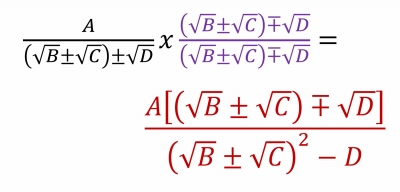Expanding the denominator: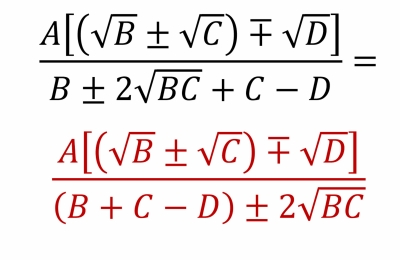Multiply by the conjugate again we get equation #7: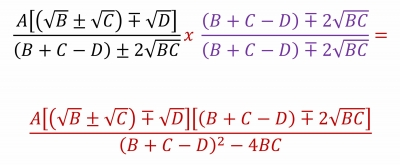As can be seen in the above seven examples, each resulting fraction becomes more and more complicated. The question that students ask is why go through all that trouble when the initial fraction appears to be simpler to use versus the resulting fraction that has been rationalized. A good questions indeed. The answer lies with precision. Before the advent of computers, all computations were done manually. The rule of thumb was to do as many “precise” computations first and then truncate the result. In most cases, performing the radical operation, results in a number that is infinitely long. If you divide a number that is infinitely long, one needs to truncate it first and then perform the division. If that same number were in the numerator then you would divide by a fixed length number into that radical term, perform the root, and then truncate the result.

That is why there was a need to rationalize the denominator. Today it has become part of mathematical convention and the irritation of millions of algebra students.

Summery equations and their multiplying identities are listed below: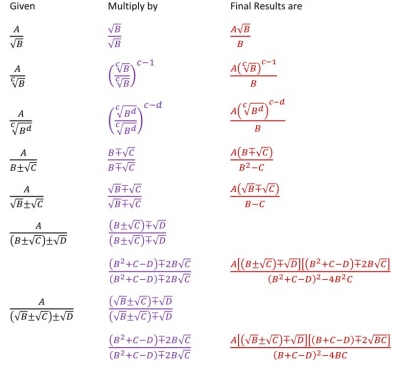If you enjoyed this mathematical derivation, you will find these articles by the same author usefu:

Deriving the Cubic Formula Detailed Steps Included

Deriving the Quadratic Formula by Completing the Square Method

How to Read and Use Roman Numerals

Estimating the Definite Integral Using Riemann Sums

The Basics on how Satellites Orbit the Earth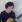Ileen Zovluck
0
Posted on Jan 5, 2011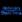Ken Brace
0
Posted on Jan 4, 2011